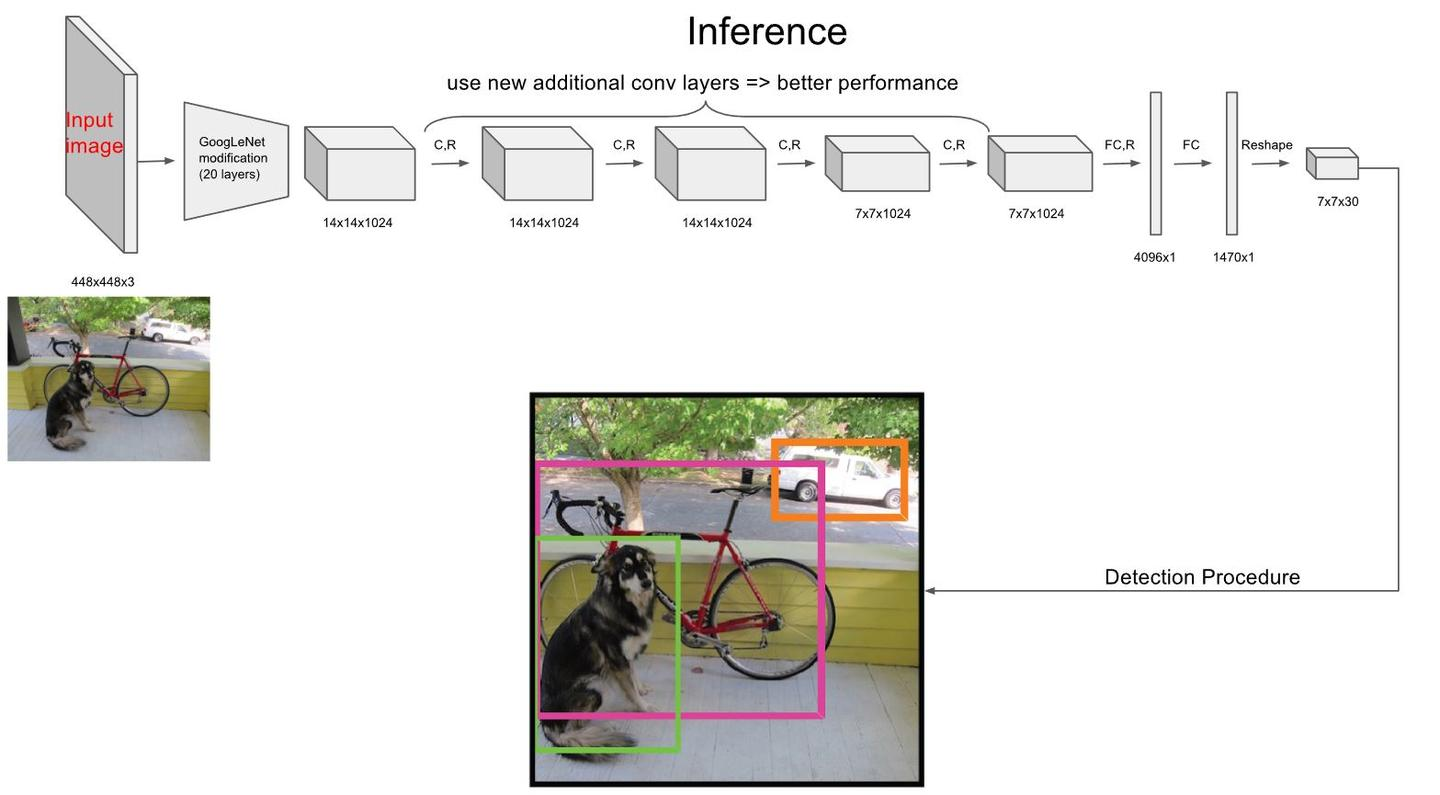## 网络设计

Yolo采用卷积网络来提取特征，然后使用全连接层来得到预测值。网络结构参考GooLeNet模型，包含24个卷积层和2个全连接层，如图8所示。对于卷积层，主要使用1x1卷积来做channle reduction，然后紧跟3x3卷积。对于卷积层和全连接层，采用Leaky ReLU激活函数：。但是最后一层却采用线性激活函数。

## 算法性能分析

• Correct：类别正确，IOU>0.5；（准确度）
• Localization：类别正确，0.1 < IOU<0.5（定位不准）；
• Similar：类别相似，IOU>0.1；
• Other：类别错误，IOU>0.1；
• Background：对任何目标其IOU<0.1。（误把背景当物体）

Yolo与Fast R-CNN的误差对比分析如下图所示：

## 算法的TF实现

Yolo的源码是用C实现的，但是好在Github上有很多开源的TF复现。这里我们参考gliese581gg的YOLO_tensorflow的实现来分析Yolo的Inference实现细节。我们的代码将构建一个end-to-end的Yolo的预测模型，利用的已经训练好的权重文件，你将可以用自然的图片去测试检测效果。

class Yolo(object):
def __init__(self, weights_file, verbose=True):
self.verbose = verbose
# detection params
self.S = 7  # cell size
self.B = 2  # boxes_per_cell
self.classes = ["aeroplane", "bicycle", "bird", "boat", "bottle",
"bus", "car", "cat", "chair", "cow", "diningtable",
"dog", "horse", "motorbike", "person", "pottedplant",
"sheep", "sofa", "train","tvmonitor"]
self.C = len(self.classes) # number of classes
# offset for box center (top left point of each cell)
self.x_offset = np.transpose(np.reshape(np.array([np.arange(self.S)]*self.S*self.B),
[self.B, self.S, self.S]), [1, 2, 0])
self.y_offset = np.transpose(self.x_offset, [1, 0, 2])

self.threshold = 0.2  # confidence scores threhold
self.iou_threshold = 0.4
#  the maximum number of boxes to be selected by non max suppression
self.max_output_size = 10

self.sess = tf.Session()
self._build_net()
self._build_detector()
self._load_weights(weights_file)

def _build_net(self):
"""build the network"""
if self.verbose:
print("Start to build the network ...")
self.images = tf.placeholder(tf.float32, [None, 448, 448, 3])
net = self._conv_layer(self.images, 1, 64, 7, 2)
net = self._maxpool_layer(net, 1, 2, 2)
net = self._conv_layer(net, 2, 192, 3, 1)
net = self._maxpool_layer(net, 2, 2, 2)
net = self._conv_layer(net, 3, 128, 1, 1)
net = self._conv_layer(net, 4, 256, 3, 1)
net = self._conv_layer(net, 5, 256, 1, 1)
net = self._conv_layer(net, 6, 512, 3, 1)
net = self._maxpool_layer(net, 6, 2, 2)
net = self._conv_layer(net, 7, 256, 1, 1)
net = self._conv_layer(net, 8, 512, 3, 1)
net = self._conv_layer(net, 9, 256, 1, 1)
net = self._conv_layer(net, 10, 512, 3, 1)
net = self._conv_layer(net, 11, 256, 1, 1)
net = self._conv_layer(net, 12, 512, 3, 1)
net = self._conv_layer(net, 13, 256, 1, 1)
net = self._conv_layer(net, 14, 512, 3, 1)
net = self._conv_layer(net, 15, 512, 1, 1)
net = self._conv_layer(net, 16, 1024, 3, 1)
net = self._maxpool_layer(net, 16, 2, 2)
net = self._conv_layer(net, 17, 512, 1, 1)
net = self._conv_layer(net, 18, 1024, 3, 1)
net = self._conv_layer(net, 19, 512, 1, 1)
net = self._conv_layer(net, 20, 1024, 3, 1)
net = self._conv_layer(net, 21, 1024, 3, 1)
net = self._conv_layer(net, 22, 1024, 3, 2)
net = self._conv_layer(net, 23, 1024, 3, 1)
net = self._conv_layer(net, 24, 1024, 3, 1)
net = self._flatten(net)
net = self._fc_layer(net, 25, 512, activation=leak_relu)
net = self._fc_layer(net, 26, 4096, activation=leak_relu)
net = self._fc_layer(net, 27, self.S*self.S*(self.C+5*self.B))
self.predicts = net

def _build_detector(self):
"""Interpret the net output and get the predicted boxes"""
# the width and height of orignal image
self.width = tf.placeholder(tf.float32, name="img_w")
self.height = tf.placeholder(tf.float32, name="img_h")
# get class prob, confidence, boxes from net output
idx1 = self.S * self.S * self.C
idx2 = idx1 + self.S * self.S * self.B
# class prediction
class_probs = tf.reshape(self.predicts[0, :idx1], [self.S, self.S, self.C])
# confidence
confs = tf.reshape(self.predicts[0, idx1:idx2], [self.S, self.S, self.B])
# boxes -> (x, y, w, h)
boxes = tf.reshape(self.predicts[0, idx2:], [self.S, self.S, self.B, 4])

# convert the x, y to the coordinates relative to the top left point of the image
# the predictions of w, h are the square root
# multiply the width and height of image
boxes = tf.stack([(boxes[:, :, :, 0] + tf.constant(self.x_offset, dtype=tf.float32)) / self.S * self.width,
(boxes[:, :, :, 1] + tf.constant(self.y_offset, dtype=tf.float32)) / self.S * self.height,
tf.square(boxes[:, :, :, 2]) * self.width,
tf.square(boxes[:, :, :, 3]) * self.height], axis=3)

# class-specific confidence scores [S, S, B, C]
scores = tf.expand_dims(confs, -1) * tf.expand_dims(class_probs, 2)

scores = tf.reshape(scores, [-1, self.C])  # [S*S*B, C]
boxes = tf.reshape(boxes, [-1, 4])  # [S*S*B, 4]

# find each box class, only select the max score
box_classes = tf.argmax(scores, axis=1)
box_class_scores = tf.reduce_max(scores, axis=1)

# filter the boxes by the score threshold

# non max suppression (do not distinguish different classes)
# box (x, y, w, h) -> box (x1, y1, x2, y2)
_boxes = tf.stack([boxes[:, 0] - 0.5 * boxes[:, 2], boxes[:, 1] - 0.5 * boxes[:, 3],
boxes[:, 0] + 0.5 * boxes[:, 2], boxes[:, 1] + 0.5 * boxes[:, 3]], axis=1)
nms_indices = tf.image.non_max_suppression(_boxes, scores,
self.max_output_size, self.iou_threshold)
self.scores = tf.gather(scores, nms_indices)
self.boxes = tf.gather(boxes, nms_indices)
self.box_classes = tf.gather(box_classes, nms_indices)